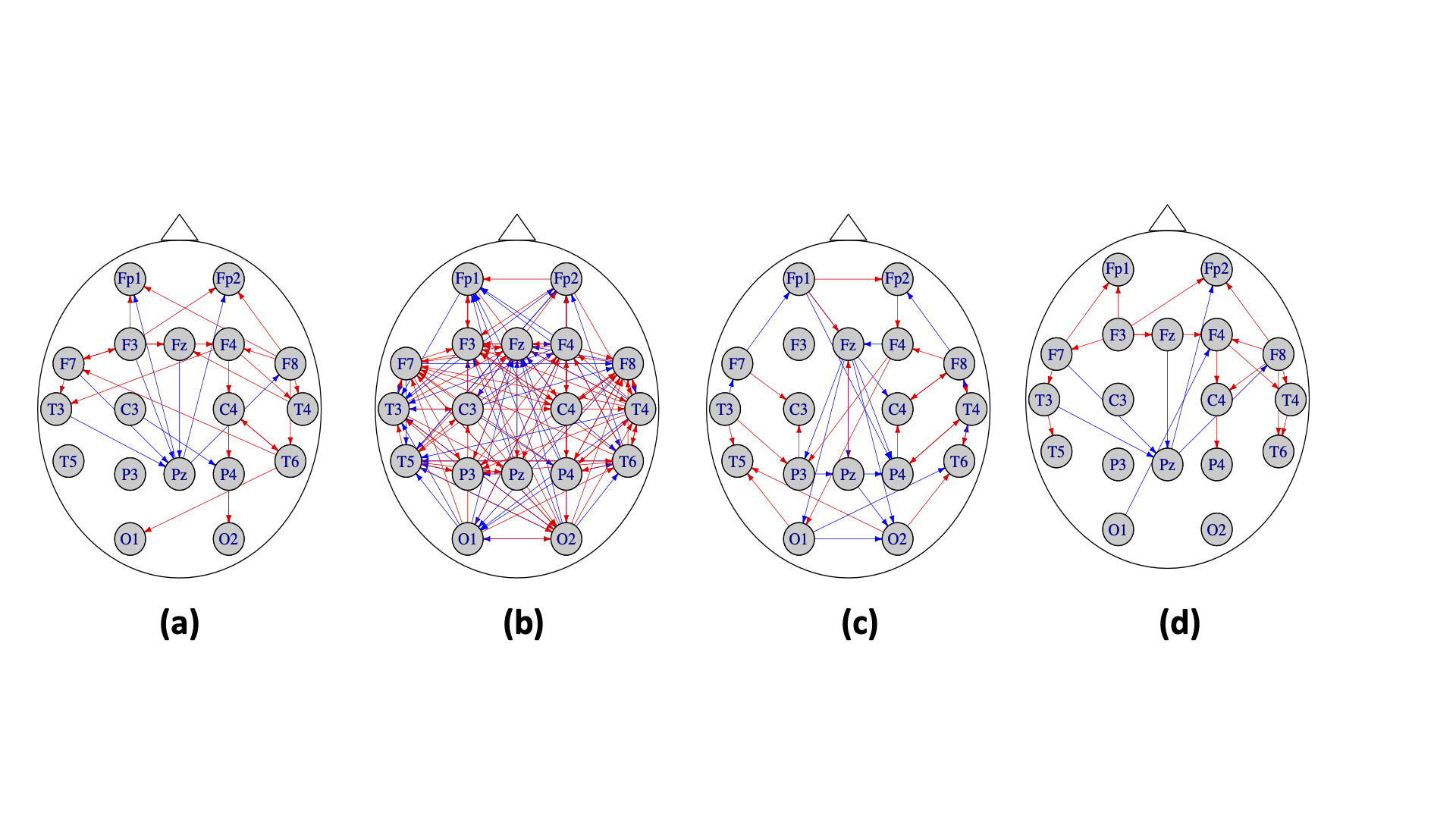Direct estimation of differential Granger causality between two high-dimensional time series

Yue Wang, Jing Ma, Ali Shojaie (2021). arXiv
ArXiv.org
Share
tweet

# Abstract

Differential Granger causality, that is understanding how Granger causal relations differ between two related time series, is of interest in many scientific applications. Modeling each time series by a vector autoregressive (VAR) model, we propose a new method to directly learn the difference between the corresponding transition matrices in high dimensions. Key to the new method is an estimating equation constructed based on the Yule-Walker equation that links the difference in transition matrices to the difference in the corresponding precision matrices. In contrast to separately estimating each transition matrix and then calculating the difference, the proposed direct estimation method only requires sparsity of the difference of the two VAR models, and hence allows hub nodes in each high-dimensional time series. The direct estimator is shown to be consistent in estimation and support recovery under mild conditions. These results also lead to novel consistency results with potentially faster convergence rates for estimating differences between precision matrices of i.i.d observations under weaker conditions than existing results. We evaluate the finite sample performance of the proposed method using simulation studies and an application to electroencephalogram (EEG) data.

Keywords: Differential Granger causality; high-dimensional time series; vector autoregression; Yule-Walker equation.